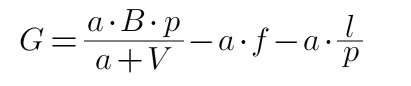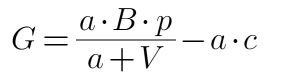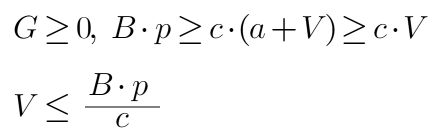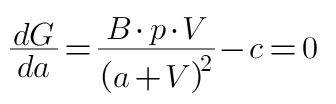# FMEX trading unlocks the optimal order volume optimization

Author: , Created: 2020-07-03 15:55:46, Updated:

FMEX’s shutdown has entrapped a lot of traders, it recently came up with a restart plan, and developed rules similar to the original “trading is mining” for unlocking debt. Trading unlocking is rather complicated. This article will give an order plan for judgment When there is profit, and the optimal order quantity. Although people should not step into the same pit twice, those who have claims on FMEX may wish to refer to the specific real market strategy.

The daily trading unlock limit will be calculated in two parts and will be refunded the next day after the total is calculated. Each part returns 50% of the trading’s daily quota separately. The specific algorithm is:

## The calculation method of the refund amount of the trading unlock amount that the user can obtain on the day of a trading (Part 2) is:

Define that every minute of each day is a trading unlocking cycle, and each cycle allocates 1/2880 of the trading unlocking limit for the day’s trading. Within each cycle, the refund amount of the unlocking cycle of the trading is allocated according to the proportion of the user’s trading volume.

The sum of the amount of credit refunded by the user for each unlock period of the trading in the trading, that is, the refund of the unlock quota available to the user on the day of the trading pair.

The first part is settled on a daily basis and cannot be calculated in advance. Here we will mainly optimize the second part, which is the minute trading unlock cycle.

# Unlock revenue from minute tradings

According to the rules, the proportion of the user’s unlocked quota in each period is equal to the proportion of the user’s trading volume in that period, and the cost includes transaction fees, loss of closing positions, etc. Obviously, within the minute period, you can’t expect a pending order to be completed. The transaction fee needs to be calculated according to the order. If you sell it backhand immediately after the trading, there will be a \$0.5 loss in closing the position (FMEX minimum pending order price change). The calculation here does not consider closing the position immediately, waiting for the next cycle to close the position first.

The unlocked earnings per minute can be obtained using the following formula:Among them, G is the unlocking gain, a is the amount of the order placed, B is the total amount of BTC unlocked in the cycle, p is the price of BTC, V is the transaction volume in the cycle, f is the transaction fee, and l is the expected loss of closing the position.

The loss of the transaction is unified as c, and the formula is simplified as:Obviously, the larger the periodic transaction volume V, the harder it is to unlock. Let us consider the following first. When V is less than, mining is advantageous:Assuming that the total value of BTC unlocked in a cycle is \$100, and the average cost is 50,000, when V is greater than \$20,000, there is no profit in transaction mining (the first part of the return is not considered)

# Optimal order quantity optimization

Since the amount of unlocking depends on the proportion of volume, if you only place an order of 1 USD, you will unlock very little. If you place an order of 100,000 USD, the cost will be very high, and you may lose money. There is an optimal order volume during the period. Direct derivation, the derivative is 0 that is to find the optimal order quantity a (a less than 0 means no order):Similarly, assuming that the total value of BTC unlocked in the cycle is 100 US dollars, that is, B*p=100, the transaction cost c=0.0005, when the cycle transaction volume V=1000, the solution has the most order amount a=13142 US dollars, and will unlock G=79.2 USD. If the cost is c=0.001, then a=9000 and G=77. You may wish to verify whether the G of other transaction volume is smaller than the optimal value.

When V=10000 and c=0.0005, a=34721 and G=28. It can be seen that as the trading volume in the cycle increases, our order volume also increases, and the return decreases.

In special cases, when V=0, a=1 (minimum order amount).

# Real market issues

The biggest problem is that I don’t know what the volume of each cycle will be. We want to place an order in the last 1s. Certainly many people will also wait for the last second to place an order, which will interfere with the calculation. The firm can be optimized according to the specific situation, such as taking into account the amount of the last order, or the custom cycle is half of the previous cycle plus half of the current cycle, do not grab the last second, etc.

There are many people who are willing to lose money to unlock and can set the transaction cost c to be lower than the actual cost.

If you want to close your position immediately after placing an order, the transaction volume will be halved, and the cost c will be the transaction fee plus 2.5% of 10,000.

More